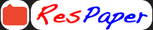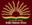Trending ▼   ResFinder# CBSE Class 12 Board Exam 2021 : Mathematics

6 pages, 75 questions, 0 questions with responses, 0 total responses,00A1 Sai Ishaan Balaji Kendriya Vidyalaya (KV), Ashok Nagar, Chennai class 12
+Fave Message
 Home > shiva2311 >Formatting page ...

CBSE Pre Board Examination 2020-21 Mathematics Class XII Max. Marks: 80 Time Allowed: 3 hrs General Instructions: 1. This question paper contains two parts A and B. Each part is compulsory. Part A carries 24 marks and Part B carries 56 marks. 2. Part-A has Objective Type Questions and Part -B has Descriptive Type Questions. 3. Both Part A and Part B have choices. Part A: 1. It consists of two sections- I and II. 2. Section I comprises of 16 very short answer type questions. 3. Section II contains 2 case studies. Each case study comprises of 5 case-based MCQs. An examinee is to attempt any 4 out of 5 MCQs. Part B: 1. It consists of three sections- III, IV and V. 2. Section III comprises of 10 questions of 2 marks each. 3. Section IV comprises of 7 questions of 3 marks each. 4. Section V comprises of 3 questions of 5 marks each. 5. Internal choice is provided in 3 questions of Section III, 2 questions of Section-IV and 3 questions of Section-V. You have to attempt only one of the alternatives in all such questions. 1. PART A Section - I Section I has 16 questions of 1 mark each. Internal choice is provided in 5 questions. Consider the set A has 5 elements, then the total number of injective functions from Set A onto  itself is ________. OR Let : is defined by ( ) = | |. Is function onto ? Give reason. 2. If R is a relation defined on set = {1, 2, 3} as = { (1, 1), (1, 2), (2,3)}. Is Relation R is  transitive, if not which ordered pairs should be added to make it transitive ? 3. The relation = {( , ): | | 3 } in set = {1, 2, 3, .10} is an equivalence  relation. Write the equivalence class related to 2. OR Let = {1, 2, 3, 4} and = {5, 6, 7}. Let : is defined as = { (1, 6), (2, 5), (3, 6), (4, 7)}. Is function bijective. Give reason. 4. If AB = 16I, then write 1 in terms of B.  5. If for matrix A, |A| = 5, find |4A|, where matrix A is of order 2 2.  OR Find the invers of matrix [7 1 ] 4 3Formatting page ...Formatting page ...Formatting page ...Formatting page ...Formatting page ...© 2010 - 2021 ResPaper. Terms of ServiceContact Us Advertise with us

ICSE Q&A - Ask and Answershiva2311 chat